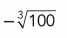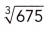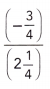# Math in Focus Grade 7 Cumulative Review Chapters 1-2 Answer Key

Go through the Math in Focus Grade 7 Workbook Answer Key Cumulative Review Chapters 1-2 to finish your assignments.

## Math in Focus Grade 7 Course 2 A Cumulative Review Chapters 1-2 Answer Key

Concepts and Skills

Write each number as $$\frac{m}{n}$$ in simplest form, where m and n are integers with n ≠ 0. (Lesson 1.1)

Question 1.
-0.87
0.87 can be written in the $$\frac{m}{n}$$ form as –$$\frac{87}{100}$$

Question 2.
12.8
12.8 can be written in the $$\frac{m}{n}$$ form as $$\frac{128}{10}$$ or $$\frac{64}{5}$$

Question 3.
-1.9
-1.9 can be written in the $$\frac{m}{n}$$ form as $$\frac{-19}{10}$$

Using long division, write each rational number as a terminating or a repeating decimal. Identify a pattern of repeating digits using bar notation. (Lesson 1.2)

Question 4.
$$\frac{9}{5}$$
Answer: $$1 . \overline{8}$$

Question 5.
$$\frac{23}{16}$$
Answer: 1.4375 it is a terminating decimal

Question 6.
–$$\frac{51}{110}$$
Answer: $$0 . 46\overline{36}$$

Use a calculator. Locate each irrational number to 2 decimal places on the number line using rational approximations. (Lesson 1.3)

Question 7.
$$\sqrt{44}$$

Question 8.
$$\sqrt{132}$$

Question 9.
–$$\sqrt{162}$$

Question 10.Question 11.
$$\frac{\pi}{7}$$

Question 12.
π3

Order the real numbers below from greatest to least using the symbol >. (Lesson 1.4)

Question 13.
$$\sqrt{345}$$, $$\frac{244}{7}$$,, –$$\frac{86}{3}$$, 33.9
Answer:$$\frac{244}{7}$$ > 33.9> $$\sqrt{345}$$>>-$$\frac{86}{3}$$

Round each number to the given number of significant digits. (Lesson 1.5)

Question 14.
349,950 (to 4 significant digits)

Question 15.
0.09608 (to 3 significant digits)

Evaluate each expression. (Lessons 2.1, 2.2)

Question 16.
7 + (-12)
7 – 12 = -5

Question 17.
-15 + (-20)
-15 – 20 = -35

Question 18.
-8 + 6 – 4
– 8 – 4 + 6
-12 + 6 = -6

Question 19.
11 – (-14)
11 – (-14)
– × – = +
11 + 14 = 25

Question 20.
32 – (-17)
32 – (-17)
– × – = +
32 + 17 = 39

Question 21.
-7 – 5 – (-6)
-7 – 5 – (-6)
-7 – 5 + 6
-12 + 6 = -6

Question 22.
-250 + 480
-250 + 480
480 – 250 = 230
The greatest value has a positive sign so put a positive sign to the obtained answer.
-250 + 480 = 230

Question 23.
-109 – (-121)
-109 – (-121)
-109 + 121
The greatest value has a positive sign so put a positive sign to the obtained answer.
121 – 109 = 12

Question 24.
43 + (-95) – (-16)
43 + (-95) – (-16)
43 – 95 + 16
43 + 16 – 95 = -36

Evaluate each product or quotient. As needed, give your answer in simplest form. (Lessons 2.3, 2.5)

Question 25.
-12.8
– × + = –
12 × 8 = 96
Put negative sign to the obtained answer.
We get -96

Question 26.
$$\frac{2}{15} \cdot\left(-\frac{5}{8}\right)$$
– × + = –
$$\frac{2}{15}$$ × –$$\frac{5}{8}$$ = –$$\frac{10}{120}$$ = $$\frac{1}{12}$$

Question 27.
$$2 \frac{2}{5} \cdot\left(-1 \frac{1}{4}\right)$$
– × + = –
2$$\frac{2}{5}$$ × -1$$\frac{1}{4}$$
$$\frac{12}{5}$$ × –$$\frac{5}{4}$$
= –$$\frac{60}{20}$$
= -3

Question 28.
-9 ÷ (-7)
Cancel – by –
9 ÷ 7 = 1.28

Question 29.
$$-\frac{7}{16} \div\left(-\frac{21}{6}\right)$$
Given,
$$\frac{7}{16}$$ × –$$\frac{21}{6}$$
= $$\frac{7}{16}$$ × –$$\frac{7}{2}$$
= –$$\frac{49}{32}$$

Question 30.–$$\frac{3}{4}$$ × 2$$\frac{1}{4}$$
= –$$\frac{3}{4}$$ × $$\frac{9}{4}$$
= – $$\frac{27}{16}$$

Evaluate each expression. (Lessons 2.3, 2.4, 2.5, 2.6)

Question 31.
-3 • 12 – (-6) + 2(-7) ÷ 7
-3 • 12 – (-6) + 2(-7) ÷ 7
((-3) 12) – (-6) + ((2 * (-7)/7))
= -32

Question 32.
$$\frac{2}{3}$$ • $$\frac{1}{6}$$ + 1$$\frac{5}{9}$$ + 2$$\left(-\frac{7}{18}\right)$$
Given,
$$\frac{2}{3}$$ • $$\frac{1}{6}$$ + 1$$\frac{5}{9}$$ + 2$$\left(-\frac{7}{18}\right)$$
$$\frac{2}{18}$$ + $$\frac{14}{9}$$ – $$\frac{43}{18}$$
– $$\frac{41}{18}$$ + $$\frac{14}{9}$$
= – $$\frac{41}{18}$$ + $$\frac{28}{18}$$
= –$$\frac{13}{18}$$

Question 33.
-4 • 5.2 – 0.5• (-7.8) + 2 • 1.3

Question 34.
–$$\frac{1}{5}$$ [-20 + 1.2(-3)] + 1$$\frac{2}{5}$$
Given,
–$$\frac{1}{5}$$ [-20 + 1.2(-3)] + 1$$\frac{2}{5}$$
–$$\frac{1}{5}$$ [-20 – 3.6] + 1$$\frac{2}{5}$$
–$$\frac{1}{5}$$ [-23.6] + 1$$\frac{2}{5}$$
4.72 + $$\frac{7}{5}$$
4.72 + 1.4
= 6.12

Problem Solving

Question 35.
An athlete completes a 400-meter dash in 46.3 seconds. What is his speed in meters per second correct to 3 significant digits? (Chapter 1)
An athlete completes a 400-meter dash in 46.3 seconds.
400/46.3
8.639
8.64 m/s

Question 36.
The greatest and least temperatures ever recorded in Tim’s hometown are 101°F and -36°F. Find the difference between these two temperatures. (Chapter 2)
Given,
The greatest and least temperatures ever recorded in Tim’s hometown are 101°F and -36°F.
101°F – (-36°F)
= 101°F + 36°F
= 137°F

Question 37.
On a math quiz consisting of 100 questions, the teacher gives 2 points for a correct answer, -1 point for an incorrect answer, and -2 points for not answering a question at all. (Chapter 2)
a) Emily answered 75 questions correctly, 18 incorrectly, and did not answer 7 questions. What is her score?
75 × 2 = 150
18 × -1 = -18
7 × -2 = -14
150 -18 – 14
150 – 32 = 118
Thus the score of Emily is 118

b) Amy answered 30 questions correctly and 62 incorrectly. What is her score?
30 × 2 = 60
62 × – 1 = -62
60 – 62 = -2
Amy score is -2

Question 38.
Justin dug a hole that was 9$$\frac{1}{2}$$ inches deep. His sister Jean built a sand castle next to the hole that was 12$$\frac{3}{4}$$ inches high. Show how you could use subtraction to find the vertical distance from the top of the castle to the bottom of the hole. (Chapter 2)
Justin dug a hole that was 9$$\frac{1}{2}$$ inches deep.
His sister Jean built a sand castle next to the hole that was 12$$\frac{3}{4}$$ inches high.
12$$\frac{3}{4}$$ – 9$$\frac{1}{2}$$
12 – 9 = 3
$$\frac{3}{4}$$ – $$\frac{1}{2}$$  = $$\frac{1}{4}$$
= 3$$\frac{1}{4}$$ inches

Question 39.
Rick started his hike at an elevation of 8,975 feet. He descended at a constant rate of 8.5 feet per minute for 45 minutes. Find Rick’s elevation after 45 minutes. Give your answer to 4 significant digits. (Chapters 1, 2)
Given,
Rick started his hike at an elevation of 8,975 feet.
He descended at a constant rate of 8.5 feet per minute for 45 minutes.
8.5 × 45 = 382.5 feet
8975 feet – 382.5 feet = 8592.5 feet
8593 feet

Question 40.
A car travels’at 68$$\frac{2}{3}$$ miles per hour for 2$$\frac{1}{12}$$ hours. Find the distance that the car has traveled correct to 3 significant digits. (Chapters 1, 2)
Answer: 68$$\frac{2}{3}$$ × 2$$\frac{1}{12}$$
= 143 $$\frac{1}{18}$$
= 143.055

Question 41.
In a particular town, the temperature at midnight is -2°C. It rises at a steady rate for 12 hours to reach a temperature of 22°C at noon. (Chapter 2)
a) Find the difference between the temperature at noon and at midnight.